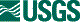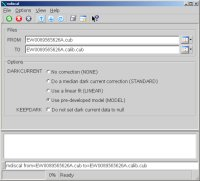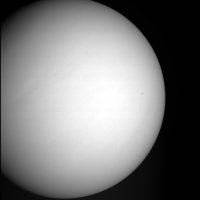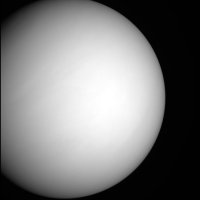## ISIS 2ISIS Application Documentation

# mdiscal

Printer Friendly View | TOC | Home

Calibrates MESSENGER/MIDS EDR/RDR products

 Overview Parameters Example 1

## Description

This program calibrates images from the Messenger mission's MDIS instrument. The input image is corrected for the following, in order, during calibration:

# Step 1: Dark current correction:

Option Description
None No dark current correction is performed.
Standard Messenger images have a strip of dark current data on the left-hand side. This will take a median of the first three sample columns (for unbinned data) and use it for the dark current on a line by line basis.
Linear This will fit a line across the first column of data, which is dark current data, and use the line to determine the dark current correction.
Model There are independent dark models, developed on-ground. This will use the most recent of these models to correct the dark current.

There are times when the user selections for dark current correction will be rejected and altered by the program. In these cases a warning message will be printed to alert the user to the change. Below is an explanation of what happens in some special cases:

DARKCURRENT parameter value Valid dark pixels exist** Exposure duration exceeds 1 second Outcome
STANDARD No No Dark current correction method is changed to MODEL. The program prints a warning message.
STANDARD No Yes Dark current correction is turned off, but the rest of the calibration is performed. The program prints a warning message.
STANDARD Yes Yes or No Dark current correction is performed with the original user input MODEL option. No warning message is needed.
LINEAR No No Dark current correction method is changed to MODEL. The program prints a warning message.
LINEAR No Yes Dark current correction is turned off, but the rest of the calibration is performed. The program prints a warning message.
LINEAR Yes Yes or No Dark current correction is performed with the original user input MODEL option. No warning message is needed.
MODEL Yes or No No Dark current correction is performed with the original user input MODEL option. No warning message is needed.
MODEL No Yes Dark current correction is turned off, but the rest of the calibration is performed. The program prints a warning message.
MODEL Yes Yes Dark current correction method is changed to STANDARD. The program prints a warning message.
** Note, there are two binning mode codes in the Instrument group of the input cube's labels, the FpuBinningMode and the PixelBinningMode. The number of valid dark pixels referred to in the table above is determined by using these values. If the sum of these two binning modes is greater than one, then there are no valid dark pixels.

# Step 2: Linearity correction:

Linearity measures the variability of a camera's DN level per unit exposure time and radiance, as a function of variation in exposure time or radiance. Using dark-corrected, desmeared DN values (DCDSI) taken from the center quarter of the images, response linearity was measured with both the NAC and WAC binned and not-binned.

# Step 3: Smear correction:

Frame transfer smear corrections for MDIS follow the technique described for NEAR MSI image by Murchie et al. (1999). In brief, an image is exposed for a nominal integration time and is then transferred in 3.84 ms to a memory zone on the CCD, from which the analog signal is digitized line-by-line. Accumulation of signal continues during the finite duration of frame transfer, inducing a streak or frame-transfer smear in the wake of an illuminated object in the field of view, parallel to the direction of frame transfer. Note that the frame transfer occurs in the calibration pixels as well as on the imaging pixels. mdiscal accounts for the frame transfer smear contributed from the 16 calibration lines (removed from the PDS-archived images).

# Step 4: Uniformity (flat field) correction:

Response uniformity, or flat field, is a measure of pixel-to-pixel variations in responsivity. Measurements of response uniformity of the WAC and NAC were conducted in the OCF, at room temperature and while cold, by imaging the integrating sphere with the two 45W bulbs illuminating the interior.

# Step 5: Absolute coefficient correction:

This converts the DN values from responsivity to radiance values.

# Step 6: Convert units to I over F:

This converts the DN values radiance values to I over F.

# Step 7: Empirical correction:

Performs an empirical correction on the DN values by scaling them using the EmpiricalCorrectionFactor. Note this correction is only applied to WAC images.

# Output units

The output units of the DNs will depend on which calibration flags are turned on.
• If IOF=TRUE, output units are measured in I/F
• Otherwise if RADIOMETRIC=TRUE, output units are measured in W/(m**2 micrometer sr)
• If RADIOMETRIC=FALSE, output units are DNs

# General calibration limitations

This program will throw an error and exit in the following circumstances:
• The input cube is multiband.
• The input cube has less than 2 samples per line.
• The input cube is not unlutted.
• PixelBinningMode > 0 and unable to reduce.

# MESSENGER MDIS calibration document excerpt

The following is an excerpt from the MESSENGER MDIS calibration document on this process

```        USAGE
=====

Raw units are of DN converted to the physical units
of radiance or I/F, following the calibration equation:

L(x,y,f,T,t,b) = Lin[DN(x,y,f,T,t,b,MET) - Dk(x,y,T,t,b,MET) -
Sm(x,y,t,b)] / {Flat(x,y,f,b) * t * [Resp(f,b,T)/Correct(f,MET)]}

where:

L(x,y,f,T,t,b) is radiance in units of W / (m**-2 microns**-1 sr**-1),
measured by the pixel in column x, row y, through filter f, at CCD
temperature T and exposure time t, for binning mode b,

DN(x,y,f,T,t,b,MET) is the raw DN measured by the pixel in column x, row
y, through filter f, at CCD temperature T and exposure time t, for binning
mode b, and Mission Elapsed Time (MET),

Dk(x,y,T,t,b,MET) is the dark level in a given pixel, derived from
a model based on exposure time and CCD temperature,

Sm(x,y,t,b) is the scene-dependent frame transfer smear for the pixel,

Lin is a function that corrects small nonlinearity of detector response,

Flat(x,y,f,b) is the non-uniformity or 'flat-field' correction,

Resp(f,b,T) is the responsivity, relating dark-, flat-, and
smear-corrected DN per unit exposure time to radiance,

Correct(f,MET) is a time-variable correction to responsivity
describing a sudden decrease in transmission of the WAC optics
on 24 May 2011 and subsequent recovery to normal values,
interpreted as due to contamination associated with MESSENGER's
first periapse season over Mercury's hot pole and the subsequent
bake-off of the contaminant,

and

t is the exposure time.

The above equation assumes that data are in the native 12-bit format in
which they were read off the CCD, and that onboard application of 12-to-8
bit lookup tables (LUTs) has been inverted.

This correction is done step-wise using the calibration tables and
images in this directory as follows.

(1) Inversion of 12 to 8 bit Compression
========================================

8-to-12 bit inversion of DN values is required when the value of
MESS:COMP12_8 is 1 (when the data are 8-bit). There are 8 inverse lookup
tables (LUTs). The table to use is indicated by the value of MESS:COMP_ALG
from 0 through 7. An 8-bit value (in a row of the table) is inverted by
replacing it with the 12-bit value in the column corresponding to a
particular LUT.

The inversion tables are given in the product MDISLUTINV_0.

(2) Subtraction of modeled dark level
=====================================

Two methods are used for subtraction of the dark level, one for
exposure times greater than 1 sec and one for exposure times less than 1 sec.

In the method for exposure times less than 1 sec, the DN values are valid
columns in the masked 'dark strip' at the edge of the CCD are fitted
linearly as a function of line in the image. Then the fits are subtracted
from the pixels in each line of the CCD.

In the method for exposure times greater than 1 sec, a model is used which accounts
for variations in column (sample) in the image, which the previous
method cannot do. However if the model were applied to longer exposures,
it would yield larger residuals than the previous method.

There are four separate models of dark level (dark current plus
electronics bias), for the MDIS-WAC and MDIS-NAC (as indicated by the
keyword INSTRUMENT_ID), and for each camera, without pixel binning turned
on (MESS:FPU_BIN = 0) or with pixel binning turned on (MESS:FPU_BIN = 1).
The models estimates the dark level Dk(x,y,t,T) as a function of column
position x, row position y, exposure time t in milliseconds (as indicated
by the keyword MESS:EXPOSURE or EXPOSURE_DURATION), and CCD temperature T
(as indicated by the keyword MESS:CCD_TEMP):

Dk(x,y,t,T) = C(T) + D(T) + [E(T) + F(T) * t] * y + {O(T) + P(T) * t +
[Q(T) + S(T) * t] * y} * x

Variables C(T), D(T), E(T), F(T), O(T), P(T), Q(T), and S(T) are all
third-order functions of CCD temperature, for example:

C(T) = H0 + H1 * T + H2 * T**2 + H3 * T**3

In all cases x or y is in the range 0-1023 for a not-binned image (as
indicated by the keyword MESS:FPU_BIN = 0) or 0-511 for a binned image (as
indicated by the keyword MESS:FPU_BIN = 1). t is in units of milliseconds,
and T is in UNCALIBRATED raw counts of CCD temperature.

For each pixel in column x and row y of an image, application of the
correction is:

DN_dark(x,y,t,T) = DN(x,y,t,T) - Dk(x,y,t,T)

where

DN(x,y,t,T) is DN in 12-bit format,

Dk(x,y,t,T) is the predicted DN level from the dark modeldark model, and

DN_dark(x,y,t,T) is dark-corrected DN.

The eight sets of coefficients for the WAC not-binned, WAC binned, NAC
not-binned, and NAC binned dark models are given in the products
MDISWAC_NOTBIN_DARKMODEL_0, MDISWAC_BINNED_DARKMODEL_0,
MDISNAC_NOTBIN_DARKMODEL_0, and MDISNAC_BINNED_DARKMODEL_0 respectively.

(3) Frame Transfer Smear Correction
===================================

Accumulation of signal continues during the finite duration
of frame transfer induces a streak or frame-transfer smear in the wake
of an illuminated object in the field of view, parallel to the direction
of frame transfer. This smear is approximated as:

Sm(x,y,t,b,f) = SUMM(1,y-1) { t2/t * [DN_dark(x,y,t,b) -
Sm(x,y,t,b,f)] / Flat(x,y,b,f)}

where

Sm(x,y,t,b,f) is the smear in column x and row y at exposure time t
in binning mode b and filter f,

Dk_dark (x,y,t,b) is dark-corrected DN in column x and row y at
exposure time t and temperature T in binning mode b,

Flat(x,y,b,f) is the flat-field correction in column x and row y in
binning mode b and filter f,

t is exposure time in milliseconds, and

t2 is the time for frame transfer (about 3.4 ms) divided by the number of
lines in the image in the direction of frame transfer, 1024 for
full-frame images (when MESS:FPU_BIN = 0) or 512 for binned images (when
MESS:FPU_BIN = 1).

For each pixel in column x and row y of an image, application of the
correction is :

DN_dark_smear(x,y,t,b,f) = DN_dark(x,y,t,b,f) - Sm(x,y,t,b,f)

where

DN_dark_smear(x,y,t,b,f) is dark- and smear- corrected DN,

DN_dark(x,y,t,b,f) is dark-corrected DN, and

Sm(x,y,t,b,f) is the smear calculated as shown above.

(4) Correction for CCD non-linearity
====================================

To remove effects of nonlinearity in WAC image data, the following
corrections should be applied after correction of dark current, bias,
and smear.

For DN_dark_smear > 1
DN_lin = DN_dark_smear/[0.008760 * Ln(DN_dark_smear) + 0.936321]

For DN_dark_smear less than or equal to 1
DN_lin = DN_dark_smear/0.936321

To remove effects of nonlinearity in NAC image data, the following
procedure should be applied after correction of dark current, bias,
and smear.

For DN_dark_smear > 1
DN_lin = DN_dark_smear/[0.011844 * Ln(DN_dark_smear) + 0.912031]

For DN_dark_smear less than or equal to 1
DN_lin = DN_dark_smear/0.912031

where

DN_dark_smear is the input dark- and smear-corrected DN, and

DN_lin is linearized dark- and smear-corrected DN.

(5) Flat-field correction
=========================

The flat field correction removes pixel to pixel differences in detector
responsivity, so that the responsivity coefficients can be expressed as
scalars for each filter. There is a separate flat-field image for MDIS-WAC
and MDIS-NAC (as indicated by the keyword INSTRUMENT_ID), without pixel
binning turned on (MESS:FPU_BIN = 0) or with pixel binning turned on
(MESS:FPU_BIN = 1), for each separate filter (as indicated by the keyword
FILTER_NUMBER). All of the files are in the 'FLAT' directory.

For each pixel in column x and row y of an image, application of the
correction is

DN_flat(x,y,f,b) = DN_lin(x,y,f,b) / Flat(x,y,f,b)

where

DN_flat(x,y,f,b) is flat-fielded, linearized, dark- and smear-corrected
DN,

DN_lin(x,y,f,b) is linearized dark- and smear-corrected DN, and

Flat(x,y,f,b) is the value in the appropriate flat-field image.

(6) Conversion from DNs to radiance
===================================

The value that relates corrected DN's measured per unit time to radiance
is the responsivity. Responsivity is modeled as a function of which camera
is being used (MDIS-WAC or MDIS-NAC as indicated by the keyword
INSTRUMENT_ID), its binning state (as indicated by the keyword
MESS:FPU_BIN), and in the case of the WAC the filter number (as indicated
by the keyword FILTER_NUMBER). The coefficients used to calculate the
responsivity are in the 'RESPONSIVITY' directory. For a camera, binning
state, and filter, the responsivity is calculated from uncalibrated CCD
temperature (as indicated by the keyword MESS:CCD_TEMP) as follows:

Resp(f,T,b) = R(f,t=-30.3C,b) * [correction_offset(f,b) + T(CCD) *
correction_coef1(f,b) + T(CCD)^2 * correction_coef2(f,b)]

where

Resp(f,T,b) is responsivity in filter f at CCD temperature T in binning
state b,

T(CCD) is raw CCD temperature in units of DNs,

R(f,t=-30.3C,b) is responsivity in filter f in binning state b at CCD
temperature of 1060 DN (-30.3C),

correction_offset(f,b) is camera- and filter-dependent temperature
correction offset for filter f and binning state b,

correction_coef1(f,b) is the camera- and filter-dependent temperature
correction first-order coefficient for filter f and binning state b.

correction_coef2(f,b) is the camera- and filter-dependent temperature
correction second-order coefficient for filter f and binning state b.

To apply responsivity to obtain radiance L, the expression is

L(f) = DN_flat(f) / (t * Resp(f,T,b) * Correct(f, MET))

where

L is radiance in units of W / (m**2 microns**1 sr**1),

DN_flat is dark-, smear-, linearity-, and flat field-corrected DN,

t is the exposure time in seconds,

Resp(f,T,b) is the responsivity in filter f at CCD temperature T and
binning state b, and

Correct(f, MET) is the temporal correction to responsivity for
filter f at time MET.

(7) Conversion from radiance to I/F
===================================

To convert from radiance to I/F (also known as radiance factor, the ratio
of measured radiance to that which would be measured from a white
perfectly Lambertian surface), the following expression should be applied:

I_over_F(f) = L(f) * pi * (SOLAR_DISTANCE/149597870.691)**2 / F(f)

where

L(f) is calibrated radiance calculated as described above for some filter
f,

SOLAR_DISTANCE is that value for distance of the target object from the
center of the sun in kilometers (as indicated by the keyword
SOLAR_DISTANCE)

149597870.691 is the number of kilometers in 1 AU

F(f) is effective average solar irradiance sampled under the filter
bandpass.

The effective average solar radiance for each camera and bandpass is given
in the SOLAR directory.
```

If run on a non-spiceinited cube, this program requires access to local mission-specific SPICE kernels, in order to find the distance between the sun and the target body. When run on a spiceinited cube, this can be determined using the camera model. Using a spiceinited cube as input has the advantage of not requiring that local mission-specific kernels be available. (See spiceinit web=true.)

## Parameter Groups

Name Description
FROM Input cube
TO Output cube

### Options

Name Description
DARKCURRENTDark current calibration method
KEEPDARKDo not set dark current data to null
FLATFIELD Apply flat field correction
IOF Convert to I/F units
ECFACTOR Apply empirical correction factor

### Files: FROM

#### Description

This is the ISIS cube from the Messenger mission to be calibrated.

 Type cube input *.cub

### Files: TO

#### Description

This is the output file. The output cube will be a radiometrically calibrated version of the input cube.

 Type cube output *.cub

### Options: DARKCURRENT

#### Description

Type string
Default MODEL
Option List:
Option Brief Description
NONENo correction No dark current correction will be applied
STANDARDDo a median dark current correction This will use the median of the three leftmost pixels as the dark current, on a line by line basis.
LINEARUse a linear fit This will fit a line to the leftmost pixel values, which should be dark current data, through the lines. The equation derived from these pixels will be used to calculate the dark current correction, on a line by line basis.
MODELUse pre-developed model Messenger has independent dark models, developed on-ground. This will use the most recent of these models to correct the dark current. Note: this method cannot be used if the exposure duration of the image exceeds one second.

### Options: KEEPDARK

#### Description

If this is selected, the dark current data are set to null in the output image.

Binning affects the number of columns that are determined to be set to null. Thus, KEEPDARK is overloaded in terms of any columns that contain artifacts that are generated by binning (or wavelet compression). These columns are set to null as well.

If no binning is applied, then four columns are set to null. If any combination of DPU or MP (essentially on-board processing) binning is applied, three columns will be set to null. When DPU and MP 2x2 binning occurs (essentially a 4x4 summing) three columns will be set to null. All other combinations of binning will have one column set to the null pixel value.

If KEEPDARK = TRUE, then no columns are set to null on output.

 Type boolean false

### Options: FLATFIELD

#### Description

This parameter allows the user to selectively apply the flatfield correction. This parameter can be used in conjunction with DARKCURRENT and RADIOMETRIC to produce additional output products such as DNs (all parameters DARKCURRENT=MODEL and RADIOMETRIC=FALSE).

 Type boolean True

#### Description

This parameter allows the user to selectively apply the radiometric correction. This parameter can be used in conjunction with DARKCURRENT and FLATFIELD to produce additional output products such as DNs (all parameters DARKCURRENT=MODEL and FLATFIELD=FALSE). However, if RADIOMETRIC=FALSE, then the program will turn IOF=FALSE.

 Type boolean True

### Options: IOF

#### Description

This option, when set, will convert the output to I/F from radiance values. To convert from radiance to I/F (also known as radiance factor, the ratio of measured radiance to that which would be measured from a white perfectly Lambertian surface), the following expression is applied:

```I_over_F(f) = L(f) * PI * (SOLAR_DISTANCE/149597870.691)**2 / F(f)
```
where L(f) is calibrated radiance calculated for some filter f, SOLAR_DISTANCE is that value for distance of the target object from the center of the sun in kilometers (as indicated by the keyword SOLAR_DISTANCE), 149597870.691 is the number of kilometers in 1 AU and F(f) is effective average solar irradiance sampled under the filter bandpass.

If the observed target is not of a valid body as recognized by NAIF, the output is left in radiance.

 Type boolean True

### Options: ECFACTOR

#### Description

This parameter is provided to allow the user to selectively apply the empirical correction.

 Type boolean True

### Example 1

Calibrating an MDIS image

#### Description

This example shows the calibration process of a MDIS image

#### Command Line

mdiscal FROM=EW0089565626A.cub TO=EW0089565626A.calib.cub DARKCURRENT=MODEL
Calibration process of a MDIS image, using the MODEL dark current option

#### GUI ScreenshotCalibrating EW0089565626A.cub Calibrate an MDIS image This is the GUI for mdiscal when converting the uncalibrated cube EW0089565626A.cub to the calibrated cube EW0089565626A.calib.cub.

#### Input ImageInput Image Input Cube This is EW0089565626A.jpg before calibration.

#### Output ImageOutput Image With Dark Model Output With Dark Model This is the output generated by the default options.